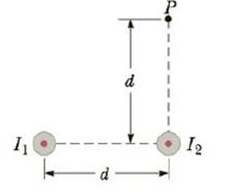Chapter 19, Problem 50P

Chapter
Section
Textbook Problem

Two long, parallel wires carry currents of I1 = 3.00 A and I2 = 5.00 A in the direction indicated in Figure P19.50. (a) Find the magnitude and direction of the magnetic field at a point midway between the wires (d = 20.0 cm). (b) Find the magnitude and direction of the magnetic field at point P, located d = 20.0 cm above the wire carrying the 5.00-A current.Figure P19.50

a)

To determine
The magnitude and direction of the net magnetic field at a point midway between the wires.

Explanation

Given info: The separation between the two wires is d=20.0cm . The current in the first wires is I1=3.00A and in the second wire is I2=5.00A . Both the wires carry current directed out of the page.

Explanation:

The arrangement of wires and points is shown in the figure.

The magnetic field due to a straight current carrying wire is given by,

B=μ0I2πr       (1)

• μ0 is the permeability of free space
• I is the current in the wire
• r is the distance of the point from the wire

Consider upward on the plane of the page to be the positive direction.

At the midpoint of the two wires the magnetic field due to the first wire will be upward on the plane of the page and due to the second wire it will be downward on the plane of the page.

Bnet=B1B2

• B1 is the magnetic field due to the first wire
• B2 is the magnetic field due to the second wire

Using (1) the net magnetic field will be given by,

Bnet=μ0I12πr1μ0I22πr2

• r1 is the distance between the point and the first wire
• r2 is the distance between the point and the second wire

The distance between the two wires and the point is d2

b)

To determine
The magnitude and direction of the net magnetic field at a point P which is 20.0cm above the second wire carrying current 5.00A .

Still sussing out bartleby?

Check out a sample textbook solution.

See a sample solution

The Solution to Your Study Problems

Bartleby provides explanations to thousands of textbook problems written by our experts, many with advanced degrees!

Get Started

White blood cells include all except the following: phagocytes killer T-cells B-cells antibodies

Nutrition: Concepts and Controversies - Standalone book (MindTap Course List)

Name the following compounds. a Cu(NO2)3 b (NH4)3P c K2SO3 d Hg3N2

General Chemistry - Standalone book (MindTap Course List)

What is motion?

An Introduction to Physical Science